# QUESTION 9 One effect on the accounting equation when a firm borrows money is that a....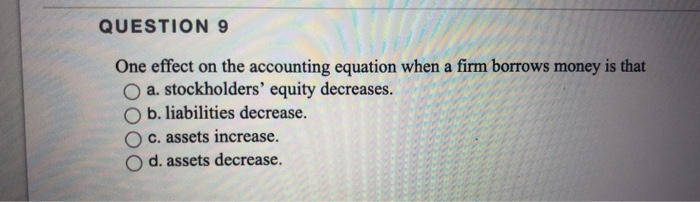QUESTION 9 One effect on the accounting equation when a firm borrows money is that a. stockholders' equity decreases. b. liabilities decrease. c. assets increase. O d. assets decrease.

This Homework Help Question: "QUESTION 9 One effect on the accounting equation when a firm borrows money is that a...." No answers yet.

We need 10 more requests to produce the answer to this homework help question. Share with your friends to get the answer faster!

0 /10 have requested the answer to this homework help question.

Once 10 people have made a request, the answer to this question will be available in 1-2 days.
All students who have requested the answer will be notified once they are available.
##### Add Answer to: QUESTION 9 One effect on the accounting equation when a firm borrows money is that a....
Similar Homework Help Questions
• ### What is the effect on the accounting equation when a company borrows \$30,000 from the bank...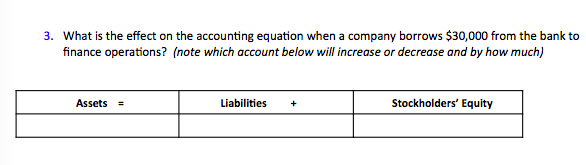What is the effect on the accounting equation when a company borrows \$30,000 from the bank to finance operations? (note which account below will increase or decrease and by how much) 3. Assets- Liabilities Stockholders' Equity

• ### When a company issues common stock for cash, what is the effect on the accounting equation...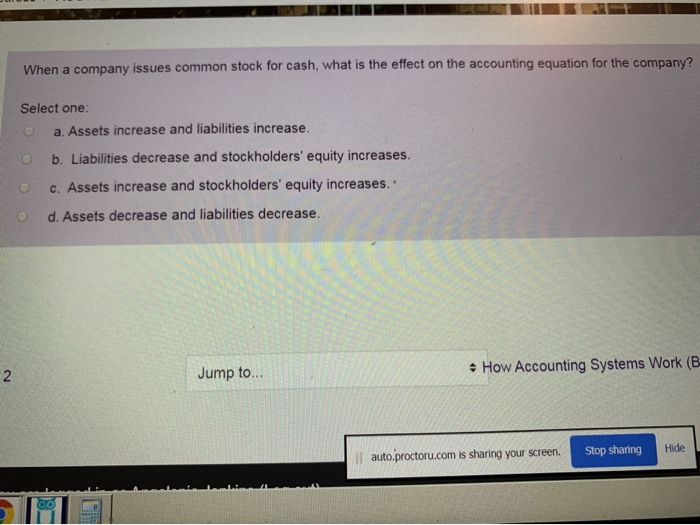When a company issues common stock for cash, what is the effect on the accounting equation for the company? Select one: a. Assets increase and liabilities increase. O b. Liabilities decrease and stockholders' equity increases. O C. Assets increase and stockholders' equity increases. O d. Assets decrease and liabilities decrease. Jump to... • How Accounting Systems Work (B. il auto.proctoru.com is sharing your screen. Stop sharing Hide

• ### Question 4 2 pts What happens to the accounting equation when the adjustment that recognizes accrued...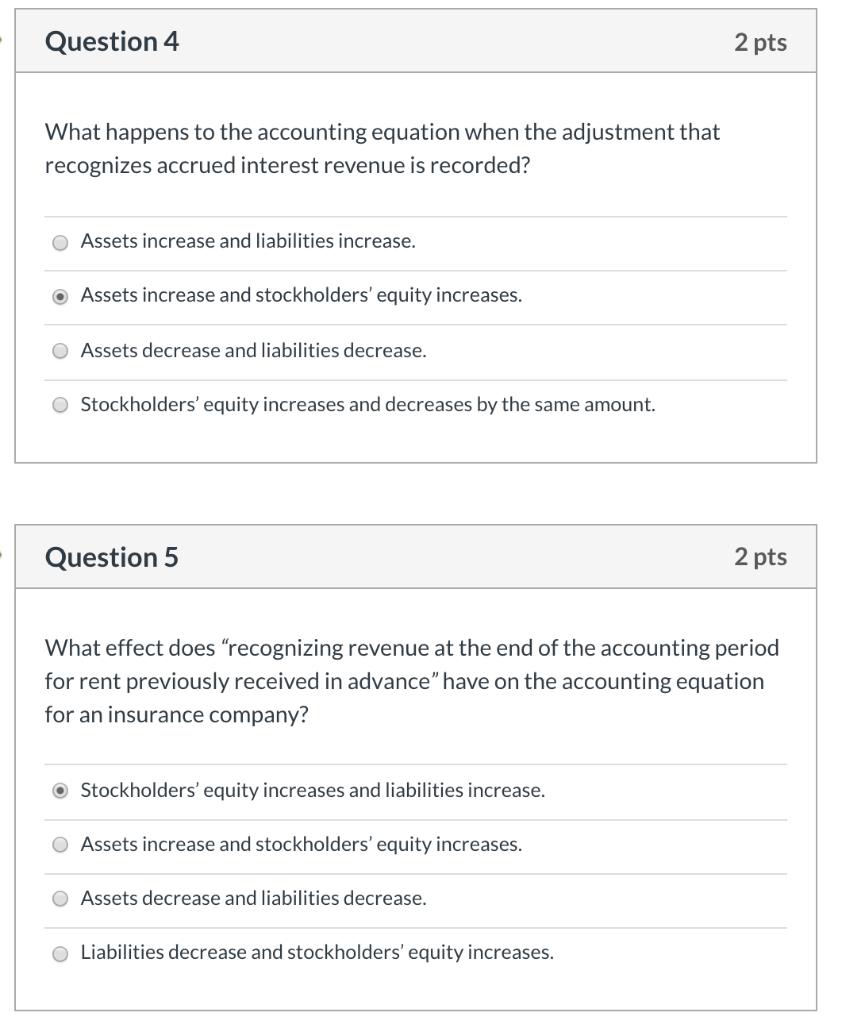Question 4 2 pts What happens to the accounting equation when the adjustment that recognizes accrued interest revenue is recorded? O Assets increase and liabilities increase. • Assets increase and stockholders' equity increases. Assets decrease and liabilities decrease. O Stockholders' equity increases and decreases by the same amount. Question 5 2 pts What effect does “recognizing revenue at the end of the accounting period for rent previously received in advance" have on the accounting equation for an insurance company? •...

• ### what effects on a retail stores accounting equation occur when merchandise return by customers who is...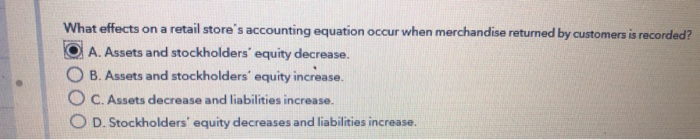what effects on a retail stores accounting equation occur when merchandise return by customers who is recorded What effects on a retail store's accounting equation occur when merchandise returned by customers is recorded? A. Assets and stockholders equity decrease. OB. Assets and stockholders' equity increase. O C. Assets decrease and liabilities increase. O D. Stockholders' equity decreases and liabilities increase.

• ### Effect of Transactions on Accounting Equation What is the effect of each of the following transactions...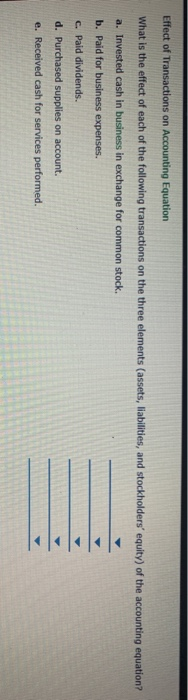Effect of Transactions on Accounting Equation What is the effect of each of the following transactions on the three elements (assets, liabilities, and stockholders' equity) of the accounting equation? a. Invested cash in business in exchange for common stock. b. Paid for business expenses. c. Paid dividends. d. Purchased supplies on account. e. Received cash for services performed. th Decreases assets and decreases liabilities. Decreases assets and decreases stockholders' equity. Increases assets and increases stockholders' equity. Increases assets and increases...

• ### If a company uses \$1,300 of its cash for Equipment, the effect on the accounting equation...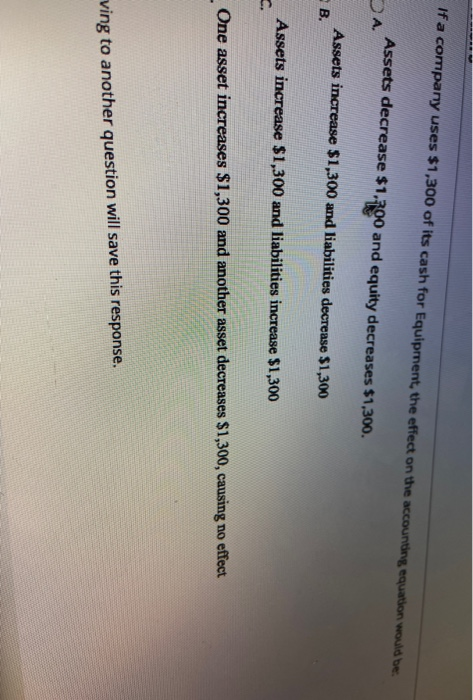If a company uses \$1,300 of its cash for Equipment, the effect on the accounting equation would be Assets decrease \$1.500 and equity decreases \$1,300. DA Assets increase \$1,300 and liabilities decrease \$1,300 B. Assets increase \$1,300 and liabilities increase \$1,300 One asset increases \$1,300 and another asset decreases \$1,300, causing no effect ving to another question will save this response.

• ### Which of the following is NOT a possible effect on the accounting equation from a single...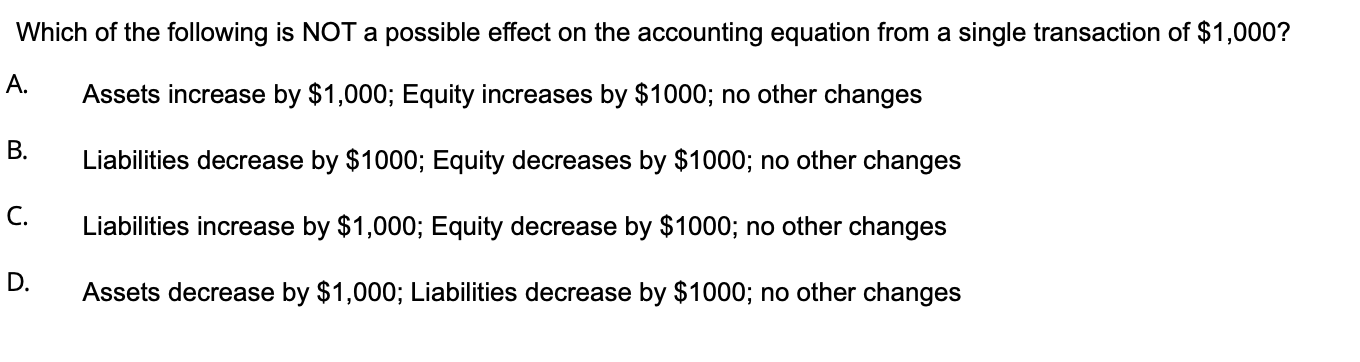Which of the following is NOT a possible effect on the accounting equation from a single transaction of \$1,000? A. Assets increase by \$1,000; Equity increases by \$1000; no other changes B. Liabilities decrease by \$1000; Equity decreases by \$1000; no other changes C. Liabilities increase by \$1,000; Equity decrease by \$1000; no other changes D. Assets decrease by \$1,000; Liabilities decrease by \$1000; no other changes

• ### Indicate how the following transactions affect the accounting equation. a. The purchase of supplies on account....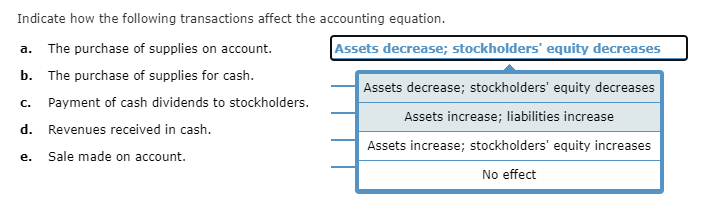Indicate how the following transactions affect the accounting equation. a. The purchase of supplies on account. Assets decrease; stockholders' equity decreases b. The purchase of supplies for cash. Assets decrease; stockholders' equity decreases Payment of cash dividends to stockholders. Assets increase; liabilities increase d. Revenues received in cash. Assets increase; stockholders' equity increases Sale made on account. No effect e.

• ### ABC Company's owner withdrawals \$2,000 cash. What is the effect on the accounting equation? A. Assets...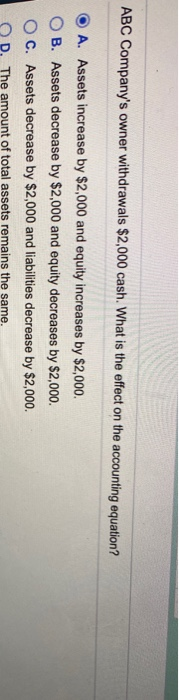ABC Company's owner withdrawals \$2,000 cash. What is the effect on the accounting equation? A. Assets increase by \$2,000 and equity increases by \$2,000. B. Assets decrease by \$2,000 and equity decreases by \$2,000. C. Assets decrease by \$2,000 and liabilities decrease by \$2,000. D. The amount of total assets remains the same

• ### Under the direct write-off method of uncollectible accounts, the effect on the accounting equation of writing...

Under the direct write-off method of uncollectible accounts, the effect on the accounting equation of writing off a customer's account is a.an increase in liabilities and a decrease in stockholders' equity (expense) b.an increase in assets and an increase in liabilities c.a decrease in assets and a decrease in liabilities d.a decrease in assets and a decrease in stockholders' equity (expense)

Free Homework App